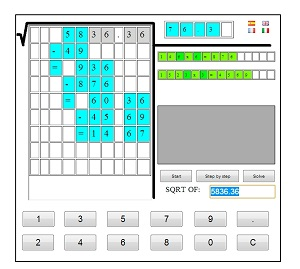# Calculate square root mentally

Calculate square root mentally

Until today I did not know Alexis Lemaire , the human calculator, but I read an article about it that surprised me so much that I have thought about putting some tricks to get calculate the square roots of head, ie mentally.
What Alexis has no name, at least to me it seems so impossible to get it and I can not imagine how has been able to calculate the thirteenth root of a 100 digit number!!! in only 3.6 seconds and the last time in the London Science Museum, calculating the thirteenth root of a 200 digit number, mentally of course, in less of 2 minutes to correctly give the result of 2,407,899,883,032,220.
But back to the techniques we use to find the square root of the head of a number.
1.- The first and most effective will be remember perfect squares, technique used by Alexis to its challenges. Obviously we will not be able to memorize so many numbers as he does, but we can start with the first and those simple as multiples of 10.
2.- Imagine now that I have memorized a few squares, for example the square of 20 is 400, but I need to calculate the square root of 484, which is near but how can I get exactly which is your square root? . So we can make use of 2 well-known formulas:$(x+1)^2 = x^2 + 2x + 1$
and$(x-1)^2 = x^2 - 2x + 1$
Mentally we know that the square of 21 is equal to the square of (20 +1 ) and according to the formula that we have seen corresponds to :$(20+1)^2 = 20^2 + 2*20 + 1 = 400 + 40 + 1 = 441$.
If we retain in head this number ( 441) we can still compute in the same way the next perfect square using the same formula:$(21+1)^2 = 21^2 + 2*21 + 1 = 441 + 42 + 1 = 484$.
Number that corresponds to the one we were looking for. Thus the square root of 484 is 22 .
3.- One last trick , if we need to calculate the square root of 16641 and it seems very large, we remove the last two digits , we are left with 166 , which is between 144 and 169 , as 169 is closer than 144 we’ll use this. The root of 169 is 13, 130 and 130 is 16900 We’re close , but as we have gone we ‘ll subtracting 130-1 apply the same formula we’ve seen. :$(130-1)^2 = 130^2 - 2*130 + 1 = 16900 - 260 + 1 = 16641$.
And there it is, our square root was 129.
Obviously not intended to reach the level of Alexis Lemaire , but perhaps this is sufficient to what we find ourselves in daily life .